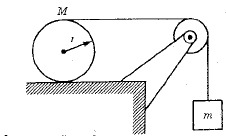# Why is T+f=Ma in Cylinder Roll Without Gliding?

• I
• Exath
In summary, the conversation is about a problem involving a cylinder rolling without gliding. The equations (1), (2), and (3) are discussed, with equation (2) being the focus. One person questions whether it should be T - f = Ma instead of T + f = Ma, as the tension and friction forces are opposite. However, it is clarified that the forces themselves act in the same direction, and it is only their torques that are opposite. The conversation ends with the realization that angular acceleration is needed in equation (3) instead of linear acceleration.

#### Exath

So I'm looking at a problem that involves a situation that looks like thisthe cylinder rolls without gliding.
And there are these following equations that apply to it
(1) mg - T = ma (for the block hanging vertically)
(2) T + f = Ma (for the cylinder f = friction force, T = String force)
(3) Tr - fr = Ia (I = inertia, r = radius)

I'm only concerned about equation (2) where it's T + f = Ma, shouldn't it be T - f = Ma? Because the tension from the string and friction force are opposite to each other. It is apparently acknowledged that they are opposite in equation (3) where the torques are opposite, but why not in equation (2)?

#### Attachments

Exath said:
I'm only concerned about equation (2) where it's T + f = Ma, shouldn't it be T - f = Ma? Because the tension from the string and friction force are opposite to each other.
Careful! The tension and friction forced are not opposite. The friction opposes slipping between surfaces, thus it acts to the right to prevent slipping of the cylinder on the table.

Exath said:
It is apparently acknowledged that they are opposite in equation (3) where the torques are opposite, but why not in equation (2)?
Since one force is above the axis and the other below, their torques are opposite. But the forces themselves act in the same direction.

•vanhees71 and Exath
Doc Al said:
Since one force is above the axis and the other below, their torques are opposite. But the forces themselves act in the same direction.

Ah, that's what i was looking for, i was looking at the forces the same way i was looking at torques, that's obviously wrong lol

Incidentally in (3) you need angular acceleration not linear acceleration.

## 1. Why is T+f=Ma in Cylinder Roll Without Gliding?

This equation represents the forces acting on a cylinder as it rolls without gliding. T is the tension force, f is the friction force, and Ma is the acceleration of the cylinder. This equation is derived from Newton's Second Law of Motion, which states that the net force acting on an object is equal to its mass multiplied by its acceleration.

## 2. What is the significance of T+f=Ma in Cylinder Roll Without Gliding?

The equation T+f=Ma is significant because it allows us to understand the relationship between the different forces acting on a rolling cylinder. By calculating the tension and friction forces, we can determine the acceleration of the cylinder and predict its motion.

## 3. How does T+f=Ma relate to the conservation of energy?

T+f=Ma is related to the conservation of energy because it takes into account the different forms of energy involved in the motion of a rolling cylinder. The tension and friction forces represent forms of potential energy, while the acceleration represents the kinetic energy of the cylinder.

## 4. What factors can affect the values of T, f, and Ma in cylinder roll without gliding?

The values of T, f, and Ma can be affected by various factors such as the mass and shape of the cylinder, the surface it is rolling on, and the presence of external forces such as wind or gravity. The coefficient of friction between the cylinder and the surface also plays a significant role in determining the values of these forces.

## 5. How can T+f=Ma be used in practical applications?

T+f=Ma can be used in various practical applications such as designing and analyzing the motion of rolling objects, such as wheels and gears. It is also used in engineering and physics to understand the forces involved in different types of motion and to make predictions about the behavior of objects in different scenarios.